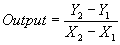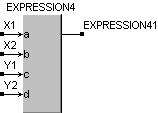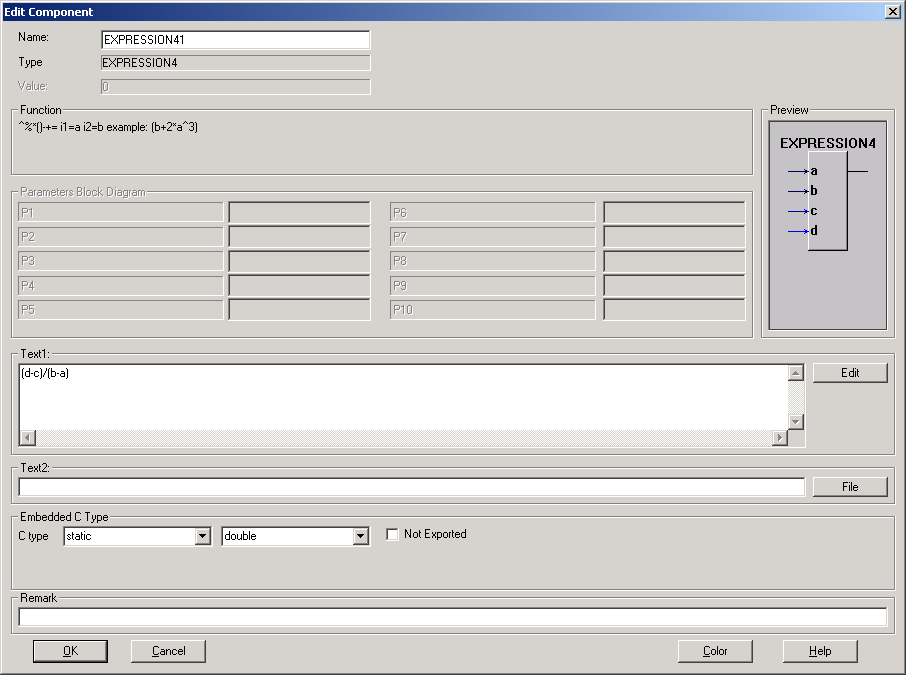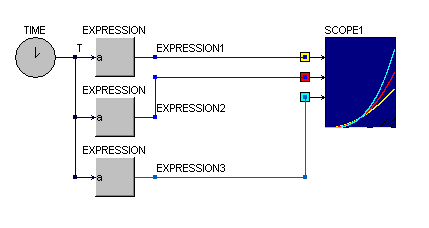• Introduction
• What is in this manual
• What is Caspoc
• User interface
• Introduction
• Starting
• Simulation
• Editing
• Viewing and printing
• Getting Started
• Basic editing
• Simulation in the time domain
• Basic User Interface Topics
• Editing
• Simulation
• Viewing
• Library
• Reports
• Project management
• Circuit and Block Diagram Components
• Introduction
• Cscript and user defined functions
• Component parameters
• Modeling Topics
• Introduction
• Power Electronics
• Semiconductors
• Electrical Machines
• Electrical drives
• Power Systems
• Mechanical Systems
• Thermal Systems
• Magnetic Circuits
• Green Energy
• Coupling to FEM
• Experimenter
• Analog hardware description language
• Embedded C code Export
• Coupling to Spice
• Small Signal Analysis
• Matlab coupling
• Tips and tricks
• Appendices

## User defined functions

In the "Expression" block a user defined function can be defined. This block is for simple algebraic equations and is faster than the Cscript block.

Depending on the number of inputs to the block, the inputs are available as a, b, c, d, ....

Use can be made of + - / * ( )

Although supported in the block "Expression", the ^ operator can be used for raising power. However it is recommended not to use the ^ operator, since it is not supported in the ANSI-C language.

To model, for example:use the following equation:

  (d-c)/(b-a) 

with following inputs:and define it at the edit field text:Always use enough brackets to structure your equations.

The user defiend function is easily inserted in your block diagram and the following example shows the comparising between

  Yellow trace = a^2 Red trace    = a^2.5 Blue trace   = a^3